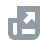Pay with
Markets
NFT
New
English
USDAccount FunctionsTutorialBinance Fan TokenBinance EarnCrypto Deposit/WithdrawalCrypto Derivatives
Futures Contracts
Introduction to Binance Futures
USDⓈ-M Futures Contracts
COIN-M Futures Contracts
Futures Guide
Cross Collateral
Hedge Mode
Types of Orders
Binance Futures Welcome Bonus Coupon
Futures Service and Agreement
Binance Futures Related Programs
Index
Portfolio Margin
Options
Leveraged TokensFinanceAPISecurityOther TopicsNFTVIP

# How to Calculate Cost Required to Open a Position in Perpetual Futures Contracts

2020-07-08 06:39
Traders should ensure that they have a minimum amount of funds in their wallet balance before opening a position. The cost required to open a position includes the initial margin and open losses (if any). Open losses occur when the price of a futures contract goes unfavorably (i.e. mark price is lower than the order price for a long order). Binance includes open losses as one of the costs required to open a position to avoid forced liquidation when traders place an order. If the open loss is not included as one of the costs required to open a position, there is a high probability that users’ position will get liquidated immediately once they have placed such order.
The formula to calculate the cost required to open a position is as such:
Cost = Initial Margin + Open Loss (if any)

## Step 1: Calculate the Initial Margin

Initial Margin
= Notional Value / Leverage
= (9,253.30 * 1 BTC) / 20
= 462.66

## Step 2: Calculate Open Loss

Open Loss
= Number of Contract * Absolute Value {min[0, direction of order * (mark price - order price)]}
direction of order: 1 for long order；-1 for short order
(i) Open loss of long order
= Number of Contract * Absolute Value {min[0, direction of order * (mark price - order price)]}
= 1 * Absolute Value {min[0, 1 * (9,259.84 - 9,253.30)]}
= 1 * Absolute Value {min[0, 6.54]}
= 1 * 0
= 0
There is no open loss when the user opens a long order.
(ii) Open loss of short order
= Number of Contract * Absolute Value {min[0, direction of order x (mark price - order price)]}
= 1 * Absolute Value {min[0, -1 * (9,259.84 - 9,253.30)]}
= 1 * Absolute Value {min[0, -6.54]}
= 1 * 6.54
= 6.54
There is an open loss when the user opens a short order.

## Step 3: Calculate the cost required to open a position

Since the long order has no open loss, thus the cost required to open a long position is equivalent to the initial margin.
(i) Cost required to open a long position
= 462.66 + 0
= 462.66
Short order has an open loss, thus the cost required to open a short position is higher as we need to take open loss into consideration besides the initial margin.
(ii) Cost required to open a short position
= 462.66 + 6.54
= 469.20 (rounding difference)

## Step 1: Calculate assuming price

Long order: assuming price = ask * (1 + 0.05%) , Open order: assuming price = max(bid, mark price)
(i) Assuming price of long order
= ask * (1 + 0.05%)
=10461.77 * (1 + 0.05%)
= 10467.0009
(ii) Assuming price of short order
= max(bid, mark price)
= max (10461.78, 10461.78)
= 10461.78
*：Level 1 price

## Step 2: Calculate the Initial Margin

Initial Margin = Notional Value / Leverage
(i) Initial margin of long order
= Assuming price of long order * Number of Contract / Leverage
= 10467.0009 * 0.2 / 20
= 104.670009
(ii) Initial margin of short order
= Assuming price of short order * Number of Contract / Leverage
= 10461.78 * 0.2 / 20
= 104.6178

## Step 3: Calculate Open Loss

Open Loss
= Number of Contract * Absolute Value {min[0, direction of order * (mark price - order price)]}
direction of order: 1 for long order；-1 for short order
(i) Open loss of long order
= Number of Contract * Absolute Value {min[0, direction of order * (mark price - order price)]}
= 0.2 * Absolute Value {min[0, 1 * (10461.78 - 10467.0009)]}
= 0.2 * Absolute Value {min[0, -5.2309]}
= 0.2 * 5.2309
= 1.04418
There is an open loss when the user opens a long order.
(ii) Open loss of short order
= Number of Contract * Absolute Value {min[0, direction of order * (mark price - order price)]}
= 0.2 * Absolute Value {min[0, -1 * (10461.78 - 10461.78)]}
= 0.2 * Absolute Value {min[0, 0]}
= 0.2 * 0
= 0
There is no open loss when the user opens a short order.

## Step 4: Calculate the cost required to open a position

Long order has an open loss, thus the cost required to open a long position is higher as we need to take open loss into consideration besides the initial margin.
(i) Cost Required to Open a Long Position
= 104.670109 + 1.04418
= 105.71 (rounding difference)
Since the short order has no open loss, thus the cost required to open a short position is equivalent to the initial margin.
(ii) Cost Required to Open a Short Position
= 104.6178 + 0
= 104.61 (rounding difference)